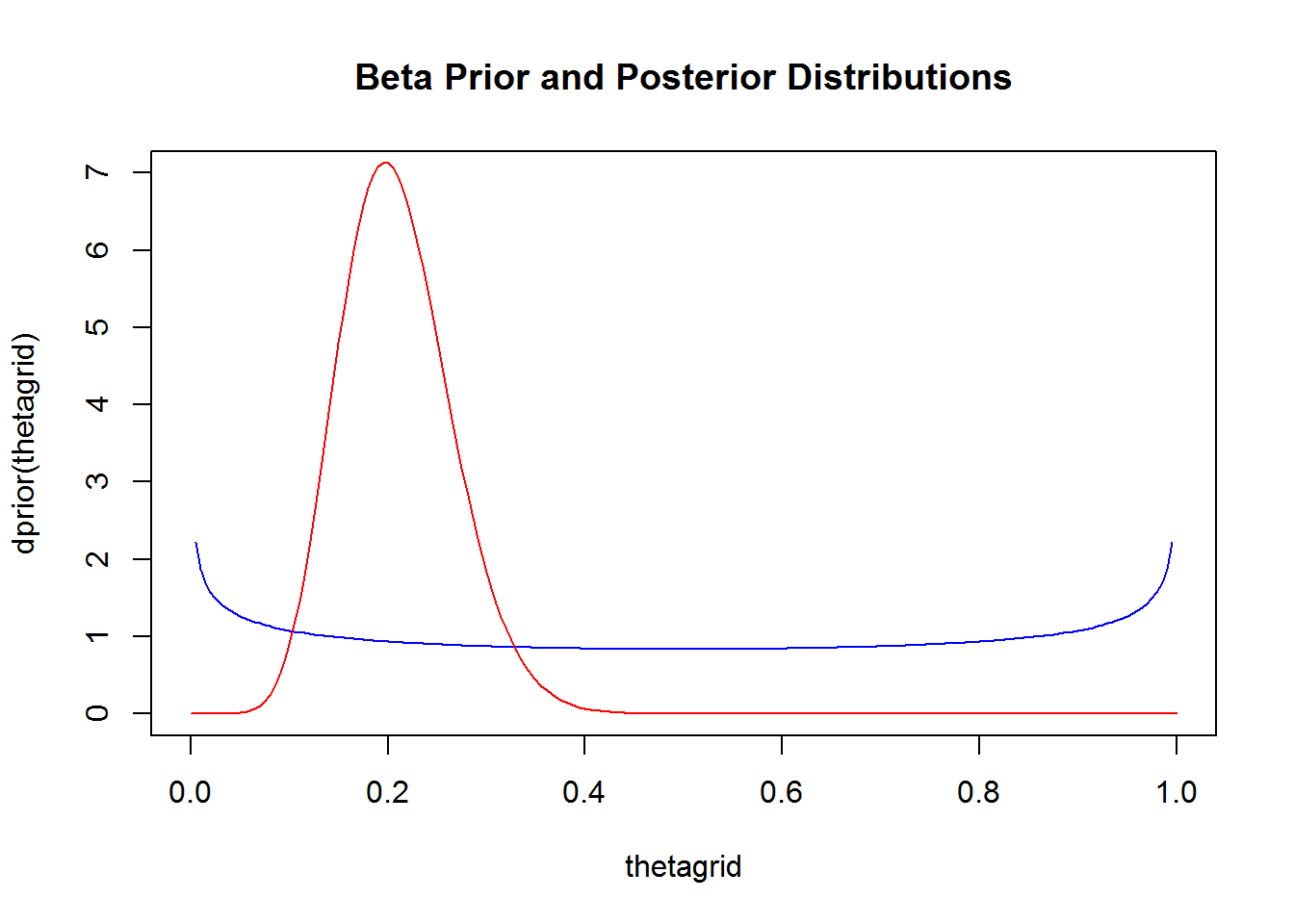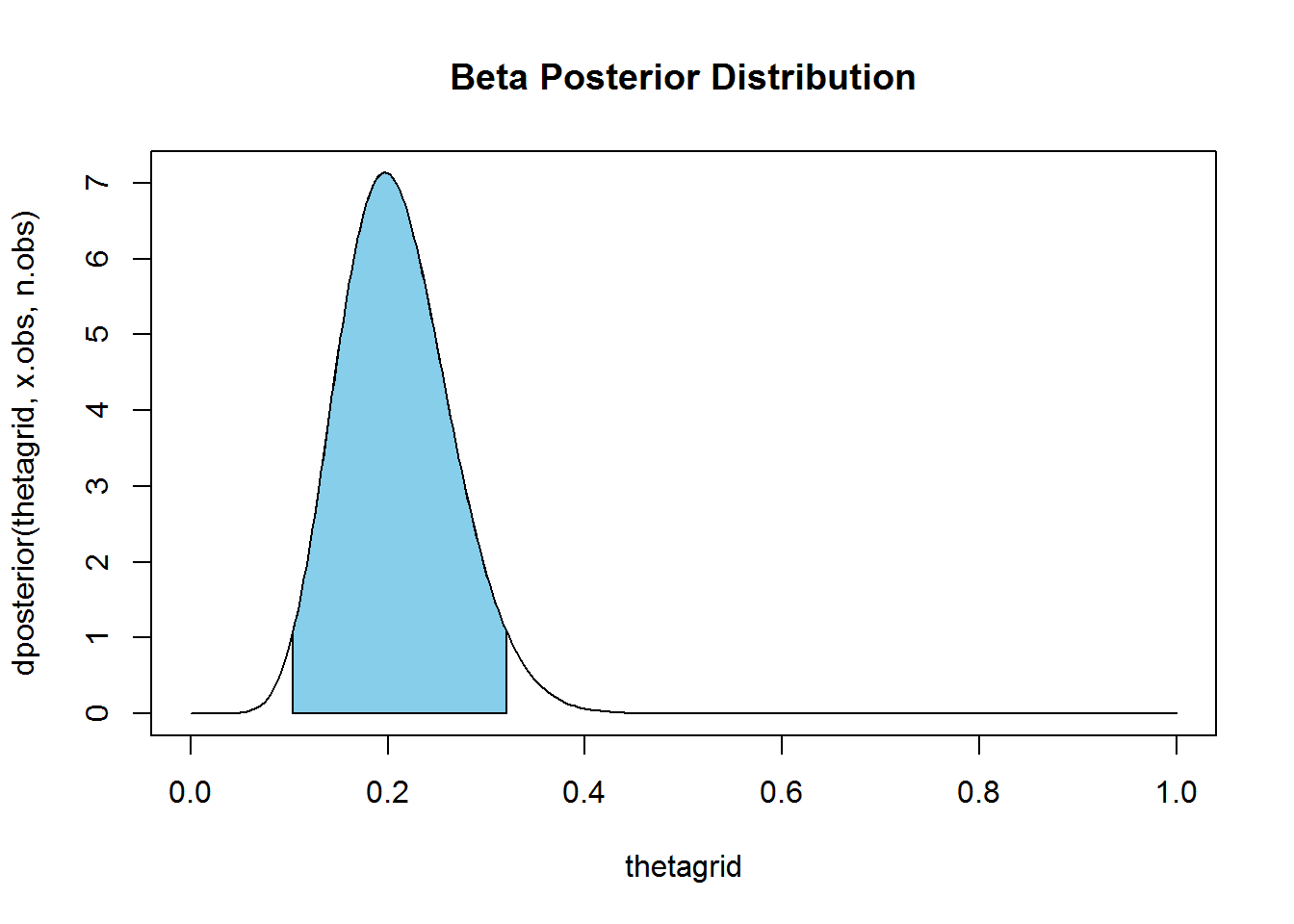# INTRODUCTION

My teaching assistant asked about coding up an example of the use of the beta prior and posterior distributions for forming a Bayesian estimate of proportion. The distributions themselves are pretty simple, and even a n00b can come up with point estimates (posterior mean, median, or mode; name your poison), but interval estimation is a bit tricky. Here’s how to it.

## Functions Used

If you are the poverbial n00b, read the helps on these to understand the trickery:

# DISTRIBUTIONS

# define the parameters
# (you could make these function arguments, your call)
# ----------------------------------------------------
alpha <- 0.75
beta  <- 0.75
# prior distribution
# ------------------
dprior <- function(theta) {
dbeta(theta, alpha, beta)
}

# posterior distribution
# ----------------------
# density
dposterior <- function(theta, x, n) {
dbeta(theta, alpha+x, beta+n-x)
}
# distribution (CDF)
pposterior <- function(theta, x, n) {
pbeta(theta, alpha+x, beta+n-x)
}
# quantile
qposterior <- function(prob, x, n) {
qbeta(prob, alpha+x, beta+n-x)
}

# ANALYSIS

## The Prior and Posterior

Plot the beta prior and posterior distributions for a sample.

n.obs <- 50
x.obs <- 10

thetagrid <- seq(from=0, to=1, length.out=201)
plot(thetagrid, dprior(thetagrid),
type="l", col="blue",
ylim=c(0,7),
main="Beta Prior and Posterior Distributions")
lines(thetagrid, dposterior(thetagrid, x.obs, n.obs),
type="l", col="red")# Highest Probability Density Region for the Posterior

Also called a credible interval, this is what passes for a confidence interval in Bayesian statistics. It’s the shortest interval that contains $$1-\alpha$$ proportion of the posterior density. Conceptually, we calculate this by lowering a horizontal line down the density curve, and finding the area under the curve between the two points where line and density curve intersect.

hpdrwidth <- function(p, prob, n, x) {
if (p+prob < 1)
w <- qposterior(p+prob, x, n) - qposterior(p, x, n)
else
w <- 1
w
}

hpdr <- function(Prob, N, X) {
L <- optimize(hpdrwidth, c(0, 1-Prob), maximum=FALSE, tol=0.00001, prob=Prob, n=N, x=X)
region <- c(qposterior(L$minimum, X, N), qposterior(L$minimum+Prob, X, N))
region
}

# calculate and plot HPDR with posterior density
# ----------------------------------------------
plot(thetagrid, dposterior(thetagrid, x.obs, n.obs), type="l", main="Beta Posterior Distribution")
R <- hpdr(0.95, n.obs, x.obs); R
##  0.1038637 0.3200036
rgrid <- seq(R, R, length.out=100)
coord.x <- c(R, rgrid, R)
coord.y <- c(0, dposterior(rgrid, x.obs, n.obs), 0)
polygon(coord.x, coord.y, col="skyblue")# test of hdprwidth optimization
# ------------------------------
L <- optimize(hpdrwidth, c(0, 0.05), maximum=FALSE, tol=0.00001, prob=0.95, n=n.obs, x=x.obs)
L
## $minimum ##  0.01675439 ## ##$objective
##  0.2161399
# ------------------------------